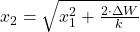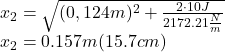Question

An ideal spring of negligible mass is 11.00 cm long when nothing is attached to it. When you hang a 3.10-kg weight from it, you measure its length to be 12.40 cm . Part A If you wanted to store 10.0 J of potential energy in this spring, what would be its total length

1.Answer: Total length is 15.7 centimeters.

Explanation:

The potential energy is modelled by applying Principle of Energy Conservation and Work-Energy Theorem: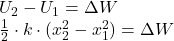The spring constant can be found by using Statics: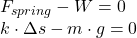Where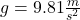.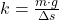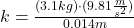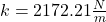The total length is isolated from energy expression: